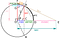# Distance between two sets of geographic coordinates (latitude, longitude) in Ruby, JS, SQL, and Python using the Haversine Formula.

In this article I’ve put together some code samples of the Haversine formula in JS, Ruby, and SQL.

# First, let me provide an explanation for the formula known as the Haversine Formula.

`The Haversine formula is perhaps the first equation to consider when understanding how to calculate distances on a sphere. The word "Haversine" comes from the function:haversine(θ) = sin²(θ/2)The following equation where φ is latitude, λ is longitude, R is earth’s radius (mean radius = 6,371km) is how we translate the above formula to include latitude and longitude coordinates. Note that angles need to be in radians to pass to trig functions:a = sin²(φB — φA/2) + cos φA * cos φB * sin²(λB — λA/2)c = 2 * atan2( √a, √(1−a) )d = R ⋅ c`https://community.esri.com/t5/coordinate-reference-systems/distance-on-a-sphere-the-haversine-formula/ba-p/902128

# SQL — Haversine Formula

`SELECT carrier.code, carrier.description, airport.lat, airport.long, ( 3959 * acos( cos( radians(airport.lat) ) * cos( radians( 61.2181 ) ) * cos( radians(149.9003) - radians(airport.long) ) + sin( radians(airport.lat) ) * sin( radians(61.2181)))) as distance_to_anchorageFROM carrierINNER JOIN airport ON airport.code=carrier.codeWHERE airport.city LIKE '%AK' AND LOWER(carrier.description) LIKE '%inc%'`
`( 3959 * acos( cos( radians(latitude1) ) * cos( radians( latitude2 ) ) * cos( radians(longitude2) - radians(longitude1) ) + sin( radians(latitude1) ) * sin( radians(latitude2))))`

# Python — Haversine

`'''Calculate distance using the Haversine Formula'''def haversine(coord1: object, coord2: object):    import math    # Coordinates in decimal degrees (e.g. 2.89078, 12.79797)    lon1, lat1 = coord1    lon2, lat2 = coord2    R = 6371000  # radius of Earth in meters    phi_1 = math.radians(lat1)    phi_2 = math.radians(lat2)    delta_phi = math.radians(lat2 - lat1)    delta_lambda = math.radians(lon2 - lon1)    a = math.sin(delta_phi / 2.0) ** 2 + math.cos(phi_1) * math.cos(phi_2) * math.sin(delta_lambda / 2.0) ** 2        c = 2 * math.atan2(math.sqrt(a), math.sqrt(1 - a))    meters = R * c  # output distance in meters    km = meters / 1000.0  # output distance in kilometers    meters = round(meters, 3)    km = round(km, 3)    print(f"Distance: {meters} m")    print(f"Distance: {km} km")‍‍‍‍‍‍‍‍‍‍‍‍‍‍‍‍‍‍‍‍‍‍‍‍‍‍‍‍‍‍‍‍‍‍‍‍‍‍‍‍‍‍‍‍‍‍‍‍‍‍‍‍‍‍‍‍‍‍‍‍‍‍‍‍‍‍‍‍‍‍‍‍‍‍‍‍‍‍‍‍‍‍‍‍‍‍‍‍‍‍‍‍‍‍‍‍‍‍‍‍‍‍‍‍‍‍‍‍‍‍‍‍‍‍‍‍‍‍‍‍‍‍‍‍‍‍‍‍‍‍‍‍‍‍‍‍‍‍‍‍‍‍‍‍‍‍‍‍‍‍‍‍‍‍‍‍‍‍‍‍‍‍‍‍‍‍‍‍‍‍‍‍‍‍‍‍‍‍‍‍‍‍‍‍‍‍‍‍‍‍‍‍‍‍‍‍‍‍‍‍‍‍‍‍‍‍‍‍‍‍‍‍‍‍‍‍‍‍‍‍‍‍‍‍‍‍‍‍‍‍‍‍‍‍‍‍‍‍‍‍‍‍‍‍‍‍‍‍‍‍‍‍‍‍‍‍‍‍‍‍‍‍‍‍‍‍‍‍‍‍‍‍‍‍‍‍‍‍‍‍‍‍‍‍‍‍‍‍‍‍‍‍‍‍‍‍‍‍‍‍‍‍‍‍‍‍‍‍‍‍‍`

# Sources

Software Developer / Follower of Jesus Christ | Yeshua the Messiah

## More from Abe Flansburg

Software Developer / Follower of Jesus Christ | Yeshua the Messiah